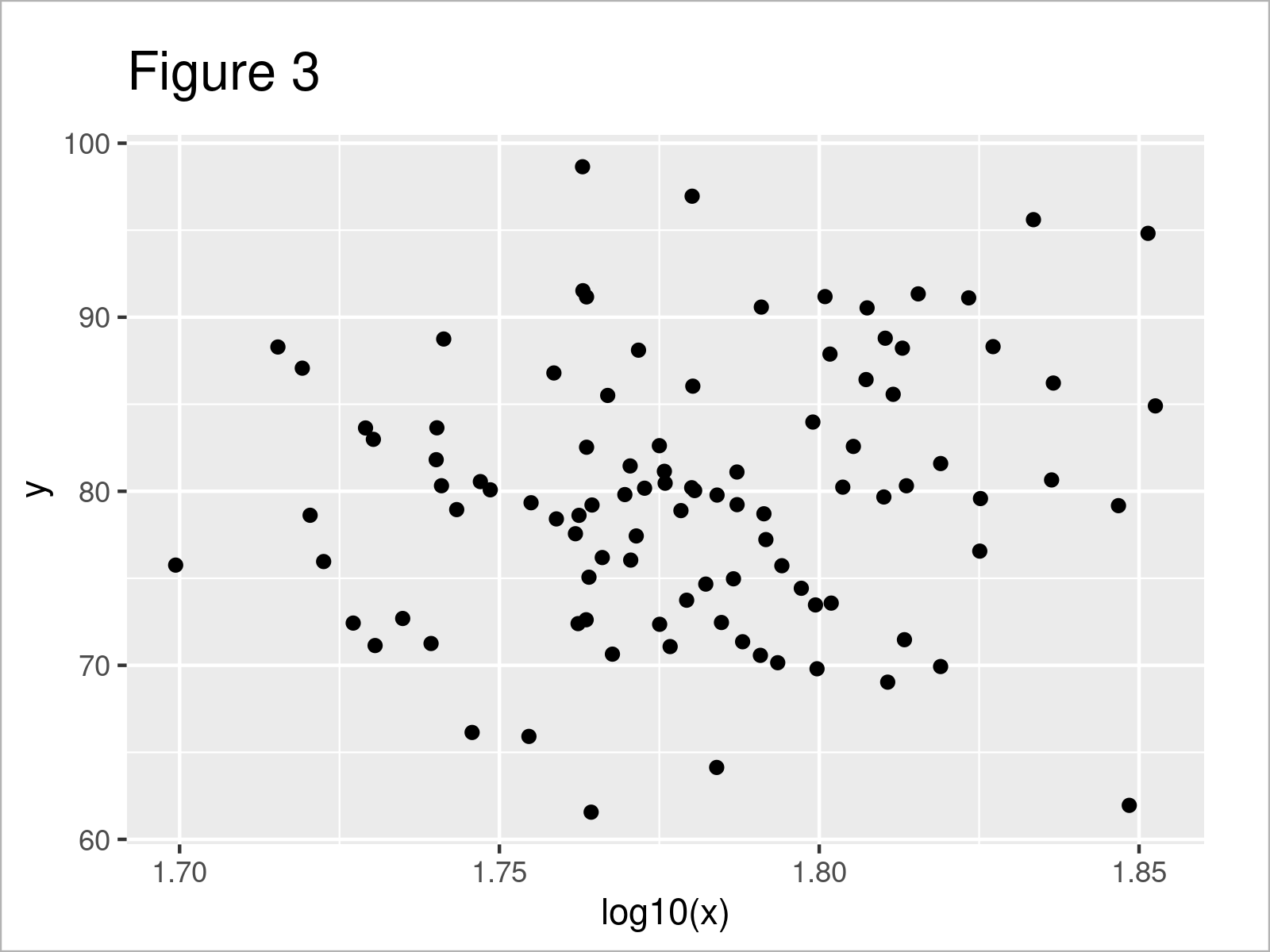# Transform ggplot2 Plot Axis to log10 Scale in R (Example)

This tutorial explains how to convert the axis of a ggplot2 graph to a logarithmic scale in the R programming language.

The content of the post is structured as follows:

Let’s jump right to the programming part:

## Example Data, Packages & Basic Plot

As a first step, we’ll need to create some data that we can use in the following examples:

```set.seed(293847)                    # Create example data
data <- data.frame(x = rnorm(100, 60, 5),
y = rnorm(100, 80, 7))
#          x        y
# 1 57.34786 86.79776
# 2 60.15527 73.74097
# 3 63.63277 80.23659
# 4 68.15397 95.60626
# 5 60.26441 80.20467
# 6 57.86862 78.61634```

As you can see based on the previous output of the RStudio console, our example data has two numeric columns.

We also have to install and load the ggplot2 package, if we want to use the corresponding functions:

```install.packages("ggplot2")         # Install & load ggplot2
library("ggplot2")```

Next, we can plot our data:

```ggp <- ggplot(data, aes(x, y)) +    # Create default ggplot2 plot
geom_point()
ggp                                 # Draw ggplot2 plot```As illustrated in Figure 1, the previous code created a ggplot2 graphic with default axis values.

## Example 1: Transform X-Axis Values of ggplot2 Plot to Logarithm with Base 10

In this example, I’ll show how to transform the values on the x-axis of our plot to a logarithmic scale with a base of 10.

For this, we have to use the scale_x_continuous function and the trans argument as shown below:

```ggp +                               # Transform x-axis to log10
scale_x_continuous(trans = "log10") +
xlab("x-log10")```As shown in Figure 2, we created a scatterplot with log scale with the previous code.

Note that we have only changed the values in our ggplot2 scatterplot. The axis tick marks have not been transformed to a log10 scale.

Depending on how you want to illustrate your data, you may prefer to show the x-axis tick marks as log10 scale as well. In the next example, I’ll therefore explain how to change the axis tick marks as well. So keep on reading!

## Example 2: Transform X-Axis Values & Axis Ticks of ggplot2 Plot to Logarithm with Base 10

In this example, I’ll illustrate how to convert the values in our plot AND the x-axis tick marks to a log10 scale.

For this, we can use the log10 function as shown below:

```ggplot(data, aes(log10(x), y)) +    # Transform x-axis to log10
geom_point()```## Video, Further Resources & Summary

I have recently published a video tutorial on my YouTube channel, which illustrates the content of this article. You can find the video below.

Please accept YouTube cookies to play this video. By accepting you will be accessing content from YouTube, a service provided by an external third party.If you accept this notice, your choice will be saved and the page will refresh.

Furthermore, I can recommend having a look at the related tutorials on www.statisticsglobe.com.